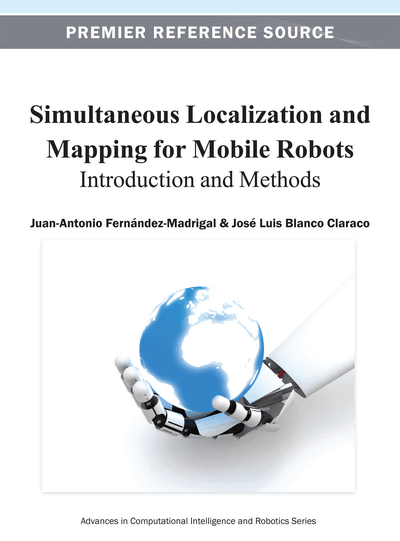# Robot Motion Models

Copyright: © 2013 |Pages: 34
DOI: 10.4018/978-1-4666-2104-6.ch005
OnDemand PDF Download:
Available
\$37.50
No Current Special Offers

## Abstract

This is the first chapter of the second section, a section devoted to mobile robot localization. Before presenting the general Bayesian framework for that problem at chapter 7, it is first required to study the different probabilistic models of robot motion. This chapter explores some of the reasons why any real robot cannot move as perfectly as planned, thus demanding a probabilistic model of the robot actions—mainly, its movements. Special emphasis is put on the most common ground wheeled robots, although other configurations (including non-robotic ones) with more degrees of freedom, such as arbitrarily-moving hand-held sensors or aerial vehicles, are also mentioned. The best-known approximate probabilistic models for robot motion are provided and justified.
Chapter Preview
Top

## Chapter Guideline

• You will learn:

• o

The particular place of odometry and proprioceptive sensors in the Bayesian filtering framework.

• o

Six different kinematic models and their corresponding motion models with uncertainty, including closed-form formulas for the case of all random variables being Gaussians.

• Provided tools:

• o

A table which relates kinematic models with physical locomotion implementations.

• o

A systematic method to obtain a probabilistic counterpart of any ideal kinematic model under the assumptions of Gaussian distributions.

• o

Practical formulas to settle hard-to-tune parameters in the constant velocity and the no-motion models.

• Relation to other chapters:

• o

Kinematic model equations are directly applicable to the particle filter methods introduced in chapters 7 and 9 for localization and map building, respectively.

• o

Probabilistic motion models for Gaussian models find their utility for localization and mapping when using parametric distributions in chapters 7, 9, and 10.

Top

## 1. Introduction

In the previous chapters, we have studied the mathematical foundations needed for addressing the problems of mobile localization and mapping. Now it is time to turn again to our robotic realm and begin to set the particular bases for solving robotics problems.

As explained in chapter 1, as a mobile robot moves throughout its environment, it constantly needs to update estimation about its instantaneous pose (i.e. the problem of localization) and, if the environment itself is unknown, a model of the world around (i.e. the problem of mapping; tackling both problems together is SLAM). In both cases the robot must rely on the readings from its sensors, both exteroceptive and proprioceptive, to perform these updates.

Both localization and SLAM consist of dynamic processes, thus we can apply recursive Bayesian estimation, introduced in chapter 4 section 9, when dealing with them. Recall that recursive here means iteratively computing the belief about some unknownsfrom the belief for the previous time step, that is,. Most probabilistic models of robot motion can be directly applied to either localization or SLAM; the only difference will be in the vector of unknowns, which in localization comprises the pose of the robot while in SLAM additionally includes a parameterized model of the world. In any case, we showed in chapter 4’s Equation 29 that the update equation for this recursive Bayesian estimator can be written as:

(1) where the transition distribution is the part of interest in this chapter.

The transition distribution is conditioned in Equation 1 to the previous state of the system. Thus,is a probabilistic function of that previous state that predicts the new state. The natural way of providingwith a mathematical form is to use some physical model of the motion of the vehicle, and the most basic of such models is the vehicle kinematics, augmented with the appropriate uncertainty. This chapter is devoted to providing some useful probabilistic models based on kinematics.

## Complete Chapter List

Search this Book:
Reset